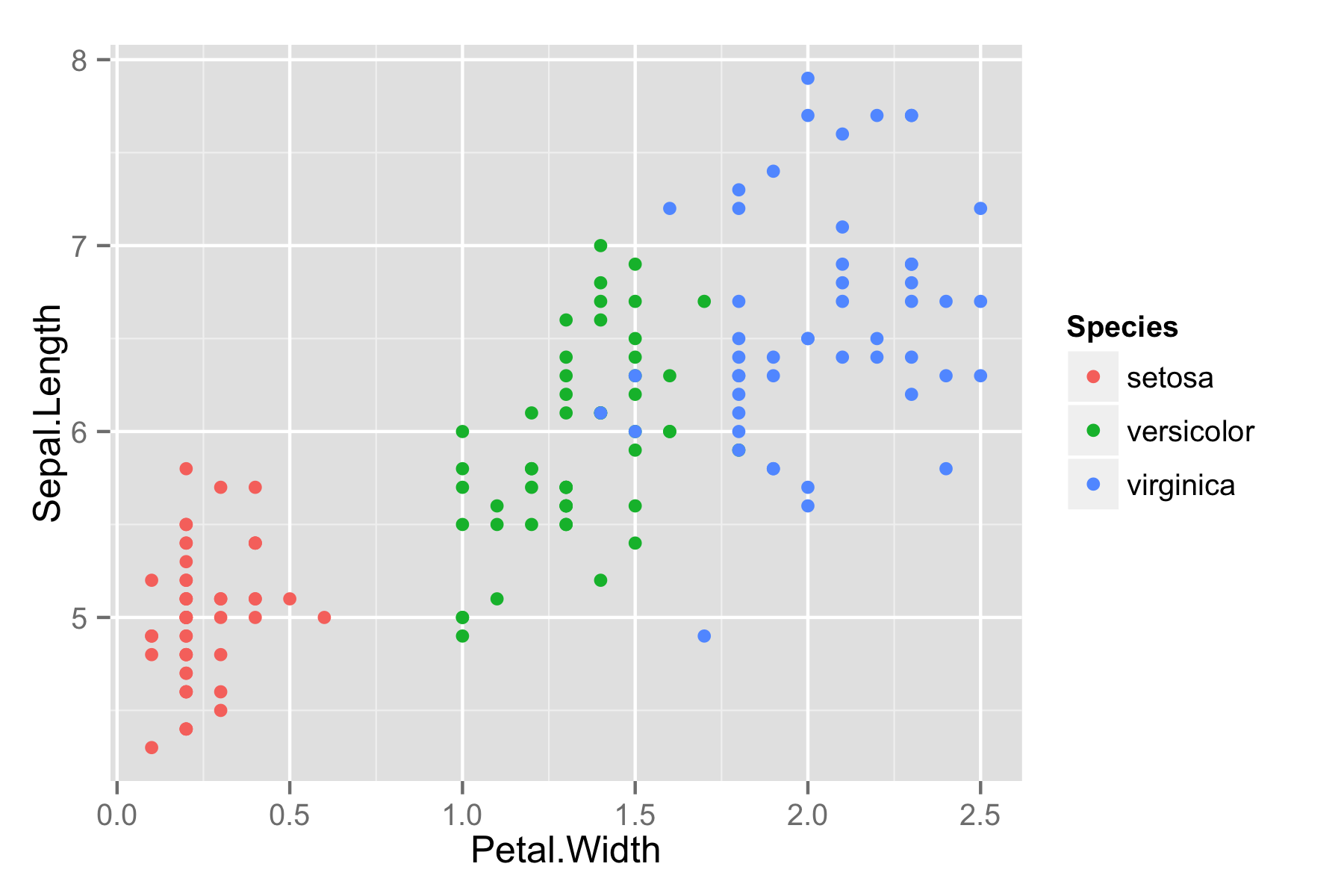rOpenSci | Make your ggplots shareable, collaborative, and with D3# Make your ggplots shareable, collaborative, and with D3

Editor’s note: This is a guest post by Matt Sundquist from Plot.ly.

Ggplotly and Plotly’s R API let you make ggplot2 plots, add `py\$ggplotly()`, and make your plots interactive, online, and drawn with D3. Let’s make some.

## 🔗 1. Getting Started and Examples

Here is Fisher’s iris data.

``````library("ggplot2")
ggiris <- qplot(Petal.Width, Sepal.Length, data = iris, color = Species)
print(ggiris)
``````Let’s make it in Plotly. Install:

``````install.packages("devtools")
library("devtools")
install_github("plotly", "ropensci")
``````

``````library("plotly")
``````
``````## Loading required package: RCurl
``````

``````signup("new_username", "[email protected]")
``````

That should have responded with your new key. Use that to create a plotly interface object, or use ours:

``````py <- plotly("RgraphingAPI", "ektgzomjbx")
``````

It just works.

``````py\$ggplotly(ggiris)
``````

The call opens a browser tab. Or in an `.Rmd` document, the plot is embedded if you specify the `plotly=TRUE` chunk option (see source). If you’re running this from the source, it makes all the graphs at once in your browser. Reaction my first time: here be dragons.

If you click the data and graph link in the embed, it takes you to Plotly’s GUI, where you can edit the graph, see the data, and share your plot with collaborators.

### 🔗 1.2 Maps

Next: Maps!

``````data(canada.cities, package="maps")
viz <- ggplot(canada.cities, aes(long, lat)) +
coord_equal() +
geom_point(aes(text=name, size=pop), colour="red", alpha=1/2, name="cities")
``````

Call Plotly.

``````py\$ggplotly(viz)
``````

### 🔗 1.3 Scatter

Want to make a scatter and add a smoothed conditional mean? Here’s how to do it in Plotly. For the rest of the plots, we’ll just print the Plotly version to save space. You can hover on text to get data, or click and drag across a section to zoom in.

``````model <- lm(mpg ~ wt + factor(cyl), data=mtcars)
grid <- with(mtcars, expand.grid(
wt = seq(min(wt), max(wt), length = 20),
cyl = levels(factor(cyl))
))

grid\$mpg <- stats::predict(model, newdata=grid)

viz2 <- qplot(wt, mpg, data=mtcars, colour=factor(cyl)) + geom_line(data=grid)
py\$ggplotly(viz2)
``````

### 🔗 1.4 Lines

Or, take `ggplotly` for a spin with the orange dataset:

``````orange <- qplot(age, circumference, data = Orange, colour = Tree, geom = "line")
py\$ggplotly(orange)
``````

### 🔗 1.5 Alpha blend

Or, make plots beautiful.

``````prettyPlot <- ggplot(data=diamonds, aes(x=carat, y=price, colour=clarity))
prettyPlot <- prettyPlot + geom_point(alpha = 1/10)
py\$ggplotly(prettyPlot)
``````

### 🔗 1.6 Functions

Want to draw functions with a `curve`?

``````eq <- function(x) {x*x}
tmp <- data.frame(x=1:50, y=eq(1:50))

# Make plot object
p <- qplot(x, y, data=tmp, xlab="X-axis", ylab="Y-axis")
c <- stat_function(fun=eq)

py\$ggplotly(p + c)
``````

## 🔗 2. A GitHub for data and graphs

Like we might work together on code on GitHub or a project in a Google Doc, we can edit graphs and data together on Plotly. Here’s how it works:

• Public use is free.
• You can set the privacy of your graph.
• You can edit and add to plots from our GUI or with R or APIs for Python, MATLA, Julia, Perl, Arduino, Raspberry Pi, and REST.
• You get a profile of graphs, like Rhett Allain from Wired Science.
• You can embed interactive graphs in iframes.

### 🔗 2.1 Inspiration and team

Plotly’s API is part of `rOpenSci` and being developed by the brilliant Toby Hocking and Plotly’s own Chris Parmer. You can find it on GitHub. Your thoughts, issues, and pull requests are welcome. Right now, you can make scatter and line plots; let us know what you’d like to see next.

The project was inspired by Hadley Wickham and the elegance and precision of `ggplot2`. Thanks to Scott Chamberlain, Joe Cheng, and Elizabeth Morrison-Wells for their help.

## 🔗 3. ggthemes and Plotly

Using `ggthemes` opens up another set of custom graph filters for styling your graphs. To get started, you’ll want to install `ggthemes`.

``````library("devtools")
install_github("ggthemes", "jrnold")
``````

``````library("ggplot2")
library("ggthemes")
dsamp <- diamonds[sample(nrow(diamonds), 1000), ]
``````

Inverse gray.

``````gray <- (qplot(carat, price, data = dsamp, colour = cut) +
theme_igray())
py\$ggplotly(gray)
``````

The Tableau scale.

``````tableau <- (qplot(carat, price, data = dsamp, colour = cut) +
theme_igray() +
scale_colour_tableau())
py\$ggplotly(tableau)
``````

Stephen Few’s scale.

``````few <- (qplot(carat, price, data = dsamp, colour = cut) +
theme_few() +
scale_colour_few())
py\$ggplotly(few)
``````
Filed under
Share
Suggest an edit Open a pull request
Browse